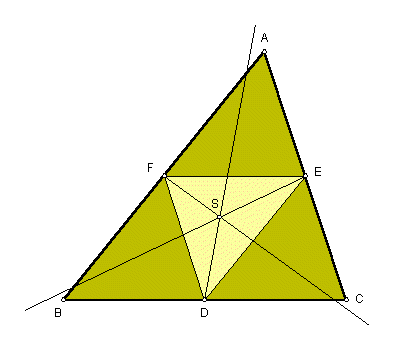# SPIEKER CENTER

 In the plane of any triangle ABC, let D = midpoint of BC, E = midpoint of CA, F = midpoint of AB; d = bisector of angle FDE, e = bisector of angle DEF, f = bisector of angle EFD. The lines d, e, f meet in a point, labeled S in the figure. Called the Spieker center, S is the center of mass of the perimeter of triangle ABC, as proved in Roger A. Johnson, Advanced Euclidean Geometry, Dover Publications, Mineola, NY, 1960, pp. 249-250.Triangle Centers